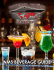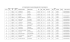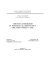# Prehomogeneousvector spaces and th elr regularity

## Transcription

Prehomogeneousvector spaces and th elr regularity
```Josai Mathematical Monographs
vol 5 (2012), pp. 71-88
Prehomogeneousvector spaces and th elr regularity
Tatsuo Kimura(a), Takeyoshi Kogiso(b), Yoshiteru Kurosawa(C),
and Masaya Ouchi(d)
Abstract
In this paper, we gather the various known constructions of prehomogeneous vector
spaces and give some new results. We consider everything over the complex number
field Åë.
Introduction
It is classically known that the Fourier transform of the complex power of the quadratic
n
form 2xi and the determinant detX of an nÅ~n matrix X are again essentially the
i==1
complex power of some po}ynomials. What is the real reason of these phenomena ? In 1961,
n
Mikio Sato realized that these polynomials 2x,2• and detX are relative invariants under
i=1
some big action of algebraic groups. Thus he reached the notion of prehomogeneous vector
spaces and showed that the Fourier transform of the complex power of a non-degenerate
relative invariant of a reductive regular prebomogeneous vector space is again essentially
the complex power of some polynomial. By using these results, one can construct the
zeta function of a regular prehomogeneous vector space which satisfies the functional
co
equations. For example, the Riemann zeta function <(s) == 21/nS can be regarded as
n==1
the zeta function of the simplest prehomogeneous vector space (GL(1),Ai,V(1)). To get
a new zeta function, a classification of prehomogeneous vector spaces is important.
For the classification of all prehomogeneous vector spaces, to find the PV-equivalences
is essential. For example, the discovery of castling transformation made the classification
of irreducible prehomogeneous vector spaces possible ([SK]). In this paper, we gather
all PV-equivalences so far known and investigate their relation of relative invariants and
regularity.
Now we give the basic definitions of prehomogeneous vector spaces and their regularity.
Let G be a linear algebraic group and p : G - GL(V) a rational representation on a
71
72
TATSUO KIMURA, TAKEYOSHI KOGISO, YOSHITERU KUROSAWA AND MASAYA OUCHI
finite-dimensional vector space V over the complex number field C. If V has a dense
G-orbit <O) = p(G)vo with respect to the Zariski topology, a triplet (G,p, V) is called
aprehomogeneous vector space (abbrev. PV). In general, an orbit p(G)vo is called an
homogeneous space. So V == p(G)vo implies that V is almost homogeneous, although it
cannot be homogeneous since p(G)O = {O}. In this sense, M. Sato named such a triplet
(G, p, V) a prehomogeneous vector space.
Then <O) is Zariski-open and its complement S is called the singular set of (G,p, V).
We call v E @ a generic point, and the isotropy subgroup at a generic point Gv == {g E
GI p(g)v = v} (v E (CD) is called a generic isotropy subgroup. Note that v E V is a
generic point if and only if dimG. = dimG- dimV. Note that (G,p, V) is a PV if and
only if (GO,plGo,V) is aPV where GO is the connected component of G. Hence for the
classification problem, we may assume that G is connected.
A non--zero rational function P(v) on V is called a relative invariant if there exists
a rational character x : G ---> GL(l) satisfying P(p(g)v) = x(g)P(v) for g E G. Let
Si = {v E SI Pi(v) = O} (i == 1,.,.,N) be irreducible components of S with codimension
one. When C is connected, these irreducible polynomials Pi,...,PN are algebraically
independent relative invariants and any relative invariant can be expressed uniquely as
P(v) = cPi(v)Mi •••PN(v)MN with cE (CX and (mi,...,mN) E ZN. These Pi,••• ,PN
are called the basic relative invariants of (G, p, V). Note that a PV (G, p, V) has no relative
invariant if and only if codimS ) 2.
By using the relations p(exptA) == exptdp(A) and x(exptA) == exptdx(A) for A E
Lie(G) and t E C, we differentiate P(p(exptA)v) = x(exptA)P(v) by t. Then we
have <dp(A)v,gp(v)> = dx(A) where gp == gradlogP : O . V* satisfies gp(p(g)v) ==
p*(g)gp(v) for g E G and v E @ . Here p" is the dual representation of p on the dual
vector space V* of V. Hence gp((Q)) is a G-orbit of the dual triplet (G,p',V'). If qp(@)
is a Zariski-dense G-orbit, we call P non-degenerate. In this case, (G,p", V*) is also a PV.
If there exists a non-degenerate relative invariant, (G,p,V) is called a regular PV. When
G is reductive, it is regular if and only if its generic isotropy subgroup is reductive.
In Section 1, we give the preliminaries for the later use.
In Section 2, we discuss the direct sum of PVs. So the classification of indecomposable
PVs will be essential.
In Section 3, we discuss the adhesion of several PVs by trivial PVs. We also discuss
the regularity.
In Section 4, we discuss the well known castling transformations and its generalization
by Y. Teranishi. We also give soine new generalization (cÅí Proposition 4.5) from which
the result of Y. Teranishi is induced.
In Section 5, we discuss Sato-Mori transformations. In this section, Proposition 5.3 is
a new result.
In Section 6, we discuss s,ymplectic PV-equiva}ence. The results about relative invariants and regularity are new.
PV'S AND THEIR REGULARITY
In Section 7, we discuss orthogonal PV-equivalence. We give the complete proof.
Notation
In general, V(n) denotes an n-dimensional vector space. If V(n) and V(n)* appear at
the same time, V(n)* denotes the dual vector space of V(n).
If O and Q appear at the same time, we write + instead of O to avoid confusion.
1 Preliminaries
All equivalences of prehomogeneity are obtained so far from the following Key Lemma.
Lemma 1.1. (Key Lemma by M. Sato)
Assu7ne that an aZgebraic group G aets on two irreducible algebraic varieties VV and
I2V'. Let g : M/ . W' be a morphism satisfying
(I? g(gbv) - gg(w) (g E G,w E W),
di? g(W) =: W'.
Then the following assertions (1? and (2? are eqzeivalent:
(1? W = G•w for some w E W,i.e., W is G-prehomogeneous.
(2? (a? W' == G•zv' foT some 2v'E W'.
(b? For the above point w' E W' in (a?, there exists a point w E g-i(zv') szcch that
g-i(w') == Gw, •w, wheTe G., = {g E G; gw' = w'} is the d,sotropy subgroup of
G at w'.
AIote that ageneric isotropy subgrozep of (1? is isomorphic to that of (2?(b? since (G.,)w ==
C.. Also note that W=G• iv i7nplies W' =G•g(w).
Proof. For the proof, see Proposition 7.6 in [K]. 1
In particular, we have the fo}lowing proposition.
Proposition 1.2. Let V and V' be finite-dimensional vector spaces on which an aZgebraic
group G acts. Assu7ne that g : V - V' is a polynomial map satisfying di q(V) == V',
(II? g(gv) = gg(v) forg E G, v E V. Then we have the folZowing assertions.
(1? If (G,V) is aPV, then (G,V') must be aPV. Moreover ifvo E V is a generic point
of (G,V), then g(vo) E V' is always a generic point of (G, V').
73
74
TATSUO KIMURA, TAKEYOSHI KOGISO, YOSHITERU KUROSAWA AND MASAYA OUCHI
(2? If P'(v') is a relative invariant of (G,V') corresponding to the character x, then
P(v) = P'(g(v)) is a relative invariant of (G,V) corresponding to the same character
X•
Proof. (1) This is a special case of the last statement of Lemma 1.1. (2) Since P(gv) =
P'(g(gv))=P'(gg(v)) =x(g)P'(g(v)) =x(g)P(v), we have our assertion. 1
Proposition 1.3. Let H be a connected algebraic group which has no nontrivial rational
eharacter, and G a connected algebraic group. Let p : ll Å~ G - GL(V) and a : G --"
GL(V') be Tational representations. Assume that there exists a sunjective polynomial map
g : V -> V' satisfying g(p(g,h)v) = a(g)g(v) (h E H,g E G,v E V). Let T : H Å~C -->
G be the projection. Assitme that (H Å~ G,p,V) is a PV with a generic point v, and
T((H Å~ G).) = Gg(.) holds. Then the basic relative invariants of (H Å~ G,p,V) are given
by {Pi,..., PN} with Pi (v) =: Pi'(g(v)) (i = 1,...,N) where {PI,..., Pft} are basic relative
invariants of (G,a, V'). If (H Å~ G,p,V) is a reductive regzLlar PV, then (G,a, V') is also
a regular PV.
PToof. See Theorem l.11 in [KKS]. Remark 1.4. In (2? of Proposition 1.2, P(v) == P'(g(v)) is a polynomial whenever P'
is a poZynomial. However even if P(v) =: P'(g(v)) is a polynomial, P' might not be a
polynomiaL For example, let G= GL(1)2 act on V= V' = C2 as V) x = (xi,x2) H
(orxi,fix2) and V' D z = (zi,z2) H (a5zi,fiz2) ((ctz,5) E G). Define g : V --ÅÄ V' by
(xi,x2) F-> (xix2,x2). Then forP(x) = xi,P'(z) = zi/z2, we have P(x) = P'(g(x)).
To prove the prehomogeneity of non-irreducible triplets, the following Proposition is
fundamental, which can be obtained immediately from the Key Lemma.
Proposition 1.5. The following assertions are eqiLivalent.
(1? (G,pi Op2, va O V2) is aPV.
(2? (a? (G,pi, Vi) is a PV with a generic isotropy subgromp H.
(b? (H, p2 IH, V2) is a PV.
2 DirectSums
We define the direct sum (Gi,m,Vi)O(G2,p2,V2) of (Gi,pi,Vi) and (G2,p2,V2) by
(Gi Å~ G2, pi X1+1X p2, Vi e V2). Similarly we can define the direct sum Oij=i(Gi, pi, Vi)
of (Gi, pi, V,) (i = 1, . . . , n).
PV'S AND THEIR REGULARITY
Theorem 2.1. (1? The direct sum O:..i(G,., pi, Vi) is a PV if and only if each component
(Gi,pi,Vi) (i= 1,...,n) is aPV.
(2? lf{Pi,...,PÅí,} are the basie reZative invariants of(Gi,p,,Vi), then
{Pii,...,Pki,,...,Pin.,...,Pkn.} are the basic retative invariants of eZ•..i(Gi,pi,Vi).
(3? The direct sum O:..i(Gi,pi, Vi) is a regiLlarPV of and only if each eomponent (Gi, p,,, Vi) (i =
1,...,n) is a regular PV.
Proof. By Proposition 1.5, we have (1). The statement of (2) is obvious. For (3), see
Proposition 1.5 in [KKTI].
1
Hence if some PVs (Gi,p,, Vi) (i = 1,...,n) are given, we can construct a new PV as
their direct sum. We say that a triplet is indecomposable if it is not the direct sum of m
triplets with m 2 2.
Lemma 3.1. Let G' be a sztbgroup of an algebraic grozLp G.
(1? (C, p, V) is a PV whenever (G', pl G, , V) is a PV.
(2? (C,p,V) has no relative invariant if(G',plG,,V) has no relative invariant.
(3? lf (G,p, V) is a regiLlar PV and (G',plG,,V) is a PV, then (G',plG,,V) is a reguZar
PV.
Proof. (1) is obvious. Since a relative invariant of (G,p, V) is a relative invariant of
(G',plG,,V), we have (2). For (3), if (G,p, V) has a non-degenerate relative invariant, it
is also a non-degenerate relative invariant of (G', plG,, V).
I
Theorem 3.2. Let p : H --> GL(m) be any rational representation of any algebraic group
H.
(1? For any n 2 m, a triplet (HÅ~ GL(n),popAi,M(m, n)) is always aPV, which is calZed
a trivial PV .
(2? Forn > m, a tTivial PV (H Å~ GL(n),p op Ai,M(m,n)) has no reZative invariant. Jn
particzelar, it is a non-regzelaT PV.
(3? Forn= m, a trivial PV (H Å~ GL(n),p C8) Ai,M(m,n)) is a regular PV.
75
76
TATSUO KIMURA, TAKEYOSHI KOGISO, YOSHITERU KUROSAWA AND MASAYA OUCHI
Proof. (1) By (1) of Lemma 3.1, we may assume that H = {e}. Define the map g : V =
M(n) -> V' =M(m,n) by X = (l]:I) H Xi. Then GL(n) acts on V= M(n) by
X H Xt24 (X E A/1(n),A E GL(n)) which is clearly a PV with a generic point I..
Hence by (1) of Proposition 1.2, a triplet ({e} Å~ CL(n),pl{.} (g> Ai,M(m,n)) is a PV
with a generic point (ImlO).
(2) By (2) of Lemma 3.1, we may assume that H = {e}. The singular set S = {X E
M(m, n) l rankX < m} is the common zeros of minors so that codim S ) 2 for n > m
and hence there is no relative invariant (see Preliminaries).
(3) If m = n, then P(X) = detX E M(n) is a non-degenerate relative invariant since
grad logdetX = tX'i for X E GL(n) (see Lemma 1.21 in [KKTI]).
1
Theorem 3.3. (Adhesion by Trivial PVs) Let p and a be rational representations of an
algebraic group G satisfying dega S n.
(1? (G Å~ GL(n),pop 1+uXAi,V (D l7V) is a PV if and only if (G,p,V) is aPV.
(2? ijdegff < n, then (GÅ~ CL(n),pX1+aopAi,VOl4i) is a non-regularPV. Note that
(G,p, V) can be a regular or a non-regular PV.
(3? ij deg a = n, then (G Å~ GL (n), pX 1 + a Q Ai, V (D I2V) is a regular PV of and only if
(G, p, V) is a regular PV.
Proof. (1) Assume that (G Å~ GL(n),pQ1+aopAi,VOW) is a PV . Then by applying
(1) of Proposition 1.2 to p : VOW . V, we know that (G, p, V) is a PV. On the other
hand, if (G,p, V) is a PV with a generic isotropy subgroup H, then by Proposition
1.5, (G Å~ GL(n),pop 1+axAi, Vol2V) is a PV if and only if (H Å~ CL(n),alHQAi, W)
is a PV. However it is a trivial PV.
(2) Ifm = dega < n, we may assume that VV = M(m,n). Then (v,X) E VOM(m,n) is
a generic point if and only if v is a generic point of (G,p, V) and rankX = m. Hence
the singu}ar set S of (G Å~ GL(n),pop 1+uopAi,VOM(m, n)) is the union {(v,X)lv E
Sv,X E M(m,n)}U{(v,X)lv E V, X E Sw} where Sv is the singular set of (G,p, V)
and Sw = {X E M(m,n)IrankX < m}. Since codim{(v,X)lv E V,X E Sw} ) 2
(see the proof of Theorem 3.2), any relative invariant on V G AII(7n,,n) is a relative
invariant on V. This implies that any relative invariant is degenerate.
(3) Since dega == n, we may assume that W == M(n). Then (v,X) E VeM(n) is a
generic point if and only if v is a generic point of (G,p,V) and rankX = n. Hence
the singular set S of (G Å~ GL(n),p (29 1+aXAi,VOM(n)) is the union {(v,X)lv E
PV'S AND THEIR REGULARITY
77
Sv,X E M(n)} U {(v,X)lv E V, detX == O}. This implies that any relative invariant
P(v,X) on VoM(n) is of the form Q(v)(det X)r ((v,X) E VOM(n)) for some integer
r where Q(v) is a relative invariant of (G,p,V). Hence we have gradlogP(v,X) =
(grad}ogQ(v),O) + (O,r tXH') E V* OM(n) ((v,X) E V OM(n) X S). This implies
that P(v,X) is non-degenerate if and only if Q(v) is non-degenerate and r l O. Thus
we obtain our assertion.
1
4 Castling Transformation and its Generalization
As we see in the previous section, (H Å~ GL(n),a op Ai,V C9 V(n)) is always a PV
if m == dimV S n. In this sectien, we shall consider the case m == dimV > n. Let
Grass.(V) == {Ul U is an n-dimensional subspace of V} be the Grassmann variety. If
a : H ---> GL(V) is a rational representation of an algebraic group H, then H acts on
Grass.(V) by U H a(h)U (h E H, U E Grass.(V)). We identify VX V(n) with M(7n,n)
and put W = {X E M(m,n)jrankX == n}. Then (H Å~ GL(n),o op Ai,V op V(n)) is
a PV if and only if W is H Å~ GL(n)-prehomogeneous. For X == (xil•••ix.) E l?V, let
<X> = (Cxi +•••+Cx. be an n-dimensional subspace of CM, i.e., <X> E Grass.(V). Then
the map g : W - W' = Grass.(V) defined by X H <X> is a surjective and it satisfies
g(a(h)X`A) = a(h)g(X) (h E H, A E GL(n)). If <X> = <Y> E Grass.(V), then as a base
change, there exists a unique A E GL(n) satisfying Y == XtA, i.e., a fiber q-i(<X>) is
GL(n)-homogeneous. Hence by Key Lemma, we obtain the former part of the following
lemma.
Lemma 4.1. Assu,me that m = dimV > n ) 1. Then the following assertions are
equivalent.
(1? (H Å~ CL(n),a op Ai,VXV(n)) is a PV.
(2? The Grassmann variety Grass.(V) is H-prehomogeneoiLs by a.
Their generic isotropy subgroiLps are isomorphic.
Proof. We show the last assertion. If X E M(m,n) is a generic point, then <X> is a
generic point of Grass.(V) by Lermna 1.1. Let p : H Å~ GL(n) - H be a projection.
We show that this induces an isomorphism p : (ff Å~ GL(n))x - H<x>. For (h,A) E
(H Å~ GL(n))x, we have <a(h)X> = <a(h)X`A> == <X>, and hence h E H<x>. Conversely
for any h E H<x>, there exists a unique A E GL(n) satisfying a(h)XtA = X. This implies
that the restriction ofpto (HÅ~GL(n))x isabijection. I
Proposition 4.2. (Castling transformation)
(1? (H Å~ GL(n),a oo Ai,VXV(n)) with m = dimV > n ) 1 is a PV if and only if
(H Å~ GL(m - n),u' op Ai,V" op V(m - n)) is a PV where a* : H - GL(V*) is
78
TATSUO KIMURA, TAKEYOSHI KOGISO, YOSHITERU
OUCHI
AND MASAYA
KUROSAWA
the dual representation ofu. These triplets are called the castling transforms of each
other. Their generie isotropy subgroups are isomorphie. There exists a one-to-one
eorrespondence of their relative invariants (ef p. 68 in ISKI?.
(2? A castling transform of a regular PV is a regular PV.
Proof. (1) The bijection f : Grass.(V) -> Grass.-.(V") defined by U H Ui is Hequivariant, i.e., f(ff(h)U) = a*(h)f(U) (h E H, U E Grass.(V)). Hence Grass.(V)
is H-prehomogeneous by a if and only if Grassm-n(V") is H-prehomogeneous by a".
Hence by Lemma 4.1, we obtain our assertion.
(2) When G is reductive, it is obvious since a reductive PV is regular if and only if
a generic isotropy subgroup is reductive. However, even if it is not reductive, this
assertion holds (see Theorem 1.30 in [KKTI], [SO]).
I
Remark 4.3. (Grassmann construction) To constrzect a new PV (H Å~ GL(m - n),a" X
Ai, V*XV(m-n)) from a given PV(HÅ~GL(n),aopAi,Vc8)V(n)) with m == dimV > n ) 1
is sometimes caZZed the Grassmann construction. In particuZar, for any given PV(G,p, V),
we can construct a new PV (GÅ~GL(m-1), p* CDAi, V*XV(m-1)). Note that the diseovery
of castling transform, i.e., Crassmann construction, made the classification of irreducible
PVs possible (/SK7?•
Theorem 4.4. Assume that m > n >- 1. Then the following assertions are eq2Livalent and
their generic isotropy subgrozeps are isomorphic.
(1? (G Å~ GL (n), px 1 + u X Ai,V O M(7n, n)) is a PV.
(2?(G Å~ GL(m - n),pX1+u* X Ai,VOM(m,m- n)) is aPV.
There exists a bijective correspondence between relative invariants of (1? and (2?.
Proof. By Propositions 1.5 and 4.2, we obtain our results. For the relative invariants,
see Proposition l.16 in [KKS]. 1
Proposition 4.5. Assume that m > n+l. Let p : H - GL(m) and a : K D GL(n) be
rational representations of algebraic groor,ps H and K. Then the following assertions are
equivaZent.
a? (HÅ~ (GLo(Z) .(*K)) , pop Al, M(m,n+l)) zs apv
re? (H Å~ (CL(M o- "- l) ., fK )) , p* xAi, M(m,m- l)) zs a PV•
Moreover their generic isotropg subgroups are isomorphic.
PV'S AND THEIR REGULARITY
Proof. The GL(l+n)-part of the generic isotropy subgroup of (GL(l+n) Å~K Ai opa, M(g+
n,n)) at (9.) is (CL.(Z) ..9K)) (= Al((GLo(t) .("K)))). Hence,by proposition 1s,
(1) is equivalent to (3) (HÅ~GL(l+n) Å~K, pcs)Ai (g)1+1opAi opu, M(m, l+n)OM(l+n, n)) or-
((H Å~ K) Å~ GL(t +n), (pX1+l op u) XAi,M(m+n,l+n)) is a PV. Its castling transform
with respect to GL(l+n) is ((H Å~K) Å~ GL(m-Z), (p* op 1+1Xa*) (21) Ai,M(m+n,m-l)).
Since this is aPV if and only if (2) holds, we obtain our assertion. ll
Example 4.6. For 2m > l+ 3, (Sp(m) Å~ (GLo(l) Go*(3)) ,Ai op Al,M(2m,l+ 3)) is
a pv if and only if(sp(m) Å~ (GL(2Mi l-3) Go*(3)) ,Ai op Ai,M(2m,2m -l)) is a
PV. ActualZy they are PVs. Note that Al = Ai for Sp(m) and SO(3).
Let P(ei,...,e.) be the standard parabolic subgroup of GL(n). Note that Ai(P(ei,...,er))
{tA-iIA E P(ei, . . . , e.)} is conjugate to P(er, • • • , ei)•
As a corollary of Proposition 4.5, we obtain the new proof of the following proposition
by Y. Teranishi.
Proposition 4.7. (Parabolic transformation [T])
Assume thatm > n = ei+• • •+er ) 1. Leta : H - GL(m) be a rational representation
of an algebraic group H. Then the following assertions are equivalent.
(1? (H Å~ P(ei, . . . , e.), a op A:, M(m, n)) is a PV.
(2? (H Å~ P(m - n, e., . . . , e2), u* X A:,M(m, m - ei)) is a PV.
PToof. By Proposition 4.5, (H Å~ P(ei, . . . , er),a X AI, M(m, n))
= (H Å~ (GL6e') p(,2,f..,..)) , u(s) Al, M(m,n)) is apv if and only if
(4 x (GL(Mo - ") p(,., i. , ,,)) , a' op Al, M(7]Z,M- ei))
== (H Å~ P(m - n, er, . . • , e2), a* X A: , M(m, m- ei)) is a PV. Also see p. 141 in [T] or p.
Note that ifr = 1, then iP is a usual castling transform.
Remark 4.8. (1? In Proposition 4.Z there exists a one-to-one correspondence between
the relative invariants of (1? and (2? (see Lemma 1.4 in !Tl?.
79
80
TATSUO KIMURA, TAKEYOSHI KOGISO, YOSHITERU KUROSAWA AND MASAYA OUCHI
(2? Altho2Lgh the castling transformation keeps the regiLlarity, the parabolic transformation
does not keep the regularity in general as we see in the following example. in Example
4.6, if we zcse GL(3) instead of GO(3), then we obtain a PV (Sp(m) Å~ P(Z,3),Ai X
AI,M(2m,Z+3)). Ifl is odd, then it is a regular PV (cf. (2? of Proposition 6.3?.
However its parabolic transform is (Sp(m) Å~ P(2m -l - 3, 3), Ai QAi, M(2m, 2m - l))
which is not regztlar sinee 2m - l is odd.
5
Sato-Mori Transformation
Theorem 5.1. (M. Sato) Assume that n ) max{mi,m2}. Let p, : G ---> GL(mi) (i == 1,2)
be rational representations ofG. Then (GÅ~GL(n),piQAi+p2QAI,M(mi,n)OM(m2,n))
is a PV if and only if (G,pi C8) p2,M(mi,m2)) is a PV•
Proof. See Theorem 7.8 in [K]. 1
Corollary 5.2. A triplet (G, p, V) is a PV of and onZy of (G Å~ GL(n),pop Ai +1 op Al, VQ
V(n) + V(n)') for any natural number n satisfying dimV g n.
Proposition 5.3. Assume that n > max{mi,m2}. Let PI,...,Pk be basic reZative invariants of (G,piQp2,M(mi,m2)). Then Pi,...,PN are basic relative invariants of
(G Å~ GL(n),pi op Ai +p2 XAI,M(mi,n) oM(m2,n)) where Pi(X,Y) = P;• (X`Y) (i :
1,...,N) for (X, Y) E M(mi,n) OM(m2,n)•
Proof. The number of basic relative invariants are the same (see Proposition 1.18 in
[KKTI]). Hence it is enough to prove that Pi is irreducib}e. If net, we have Pi = QR
for some polynomials Q and R. Since the group is connected, Q and R are also relative
invariants so that there exist Q' and R' such that Pi' == Q'R'. In general, ifapolynomial
F on M(mi,n) OM(m2,n) is defined b,y F(X,Y) == F'(XtY) for some rational function
F' on M(mi,m2), then F' is also a polynomial since F([I.,IO], [`ZIO]) == F'(Z) holds.
Hence Q' and R' are polynomials, which contradicts an irreducibility of P/, (cf. Remark
Proposition5.4. lfn > max{mi,m2}, then aPV(GxGL(n),piQAi+p2XAi,M(mi,n)O
M(m2,n)) is a regiLlar PV if and only of mi == m2.
Proof. Assume that mi = m2(=: m). Then the image of G Å~ GL(n) is a subgroup of the '
image of the group of (GL(m) x GL(m) Å~ GL(n),Ai Q 1 CD Ai + 1 XAi op Af,M(m, n) e
M(m, n)). It is castling equivalent to ((GL(m) Å~ GL(n-m)) Å~ GL(n), (Ai C8) 1+1 op Ai) (29
Ai, M(n)) which is a regular trivial PV. Hence by (3) of Lemma 3.1 and Theorem 4.4, our
PV is a regular PV. If mi l m2, it is a non-regular PV by Proposition 1.22 in [KKTI].
1
PV'S AND THEIR REGULARITY
Proposition 5.5. ij (G,m op p2,M(mi,m2)) is a regular PV, then (G Å~ GL(n),pi op
Ai +p2 op Ai,M(mi,n) OM(m2,n)) with n = max{mi,m2} is a regular PV. So far, the
converse holds under some conditions, but notproved in general yet.
Proof. See (2) of Proposition 1.20 in [KKTI].
1
Proposition 5.6. Assume that n = mi ) m2. Then a relative invariant P(X,Y)of a
PV (C Å~ GL(7ni),pi op Ai +p2 op Ai,M(mi)OM(m2,mi)) is of the form P(X,Y) =
P'(XtY)(detX)r where P'(Z) is a relative invariant of (G,pi op p2,M(mi,m2)) and r E
Z. In this case, even of P'(Z) is an iTreducible polynomial, P(X,Y) = P'(.XtY) is not
necessarily irreducible.
Proof. By (2) of Proposition 1.18 in [KKTI], we have the former result. For the latter
assertion, for example, if n = mi = m2, then P'(Z) == detZ is an irreducible polynomial,
but P(X, Y) = P'(XtY) = detX det Y is not irreducible.
Theorem 5.7. The following assertions are equivalent.
(1? (G,p+pi op p2,VOM(mi,m2)) is aPV.
(2? (C Å~ GL(n),pop 1+pi XAi +p2 CD Ai,VeM(mi,n) OM(m2,n)) is a PVfor all n
satisfying n ) max{ml, m2}
ij n > max{mi,m2}, there exists a bop'ective correspondence between the relative invariants of (1? and (2?.
Proof. By Proposition 1.5 and Theorem 5.1, we obtain the equivalence of (1) and (2).
For the latter assertion, one can prove similarly as Proposition 5.3.
1
Now consider the case mi > n ) m2.
Theorem 5.8. ([KKS2])
For mi > n > m2, the following asseTtions are equivalent.
(1? (G Å~ GL (n), pi X Ai + p2 C8) Af, M(mi, n) ee M(m2, n)) is a P V.
(2? (a? (G, pi op p2, M(mi,m2)) is aPV with a generic point Zo EM(mi,m2).
(b? LetH be the isotropy subgTo?Lp ofG at Zo,p: the d2Lal action ofpi and <Zo>Å} ==
{f E Y(mi)*lf(v) - O for all v E <Zo>}.
Then (H Å~ GL(mi -n), pl op Ai, <Zo>i op V(mi - n)) is aPV.
81
82
TATSUO KIMURA, TAKEYOSHI KOGISO, YOSHITERU KUROSAWA AND MASAYA OUCHI
Moreover generic isotropy subgroups of (1? and (2?(b? are isomorphic. In particular, when
G is reduetive, (1? is a regular PV of and only if a generic isotropy subgroup of (2?(b? is
reductive.
Note that if mi > n =: m2, then (1) and (2)(a) in Theorem 5.8 are equivalent since the
generic isotropy subgroup at I. of (G Å~ GL(m2), p2 c}9 Al, M(n)) is {(A, p2(A)); A E G}.
The special case n = mi-1 and m2 = 1 of Theorem 5.8 was already known ( See Theorem
1.1 in [KUY], Theorem 7.10 in [K]) and used to prove that (GL(1)4 Å~ G, p, V((2n +
1)(2m+2n-1))) is aPV and (GL(1)5xG, p+IXAi*)op1, V((2n+1)(2m+2n-1))OV(2m))
is a non-PV where G == Sp(n) Å~ SL(2m) Å~ SL(2n - 1) and p == Ai op Ai op 1 + Ai op 1 op
Ai + 1 XAS*) X 1 + 1 op 1 op Al (See Lemmas 3.31 and 3.33 in [KUY]).
For the case min{mi,m2} ) n, we have the following result.
Let G be a linear algebraic group. Let pi : C - GL(mi) and p2 : G --> GL(m2)
be its rational representations. Then G acts on M(mi,m2) by pi op p2, i.e., X H
pi(g)Xtp2(g) (X E M(mi,m2), g E G). By this action, G also acts on a rank vari-
ety M(n)(mi,m2) = {X EM(mi,m2)l rankX == n}.
Theorem 5.9. ([OHK]) The following assertions are equivalent.
(1? M(")(mi,m2) has a Zariski-dense G-orbit by the action pi X p2.
(2? (G Å~ GL(n), pi CD Ai +p2QAi, M(mi,n) OM(m2,n)) is aPV.
Here the action of (2? is given by (X,Y) H (pi(g)X`A,p2(g)YA-') for (X,Y) E
M(mi,n) O IVI(m2,n) and (g, A) E G Å~ GL(n).
6
Symplectic PV-equivalence
Let a : H . GL(n) be arational representation ofa linear algebraic group H. Then the
action A2(a) of H on Alt(n) == {X E M(n)I tX : -X} is given by X H a(h)X`a(h) (h E
H,X E AIt(n)). Let Sp(7n) = {A E GL(2m)I `AJA = J} be the symplectic group where
J= (-9m IoM). For x,?y E (C2m, if we define <x,y> = txJy == 2:-I,l.',i(xiym+i -xm+iyi.),
we have <Ax,Ay> = <x,y> for A E Sp(m). Now we investigate the relation of 2 triplets
(Sp(m) Å~ H, Ai Q o, M(2m, n)) and (H, A2(o), Alt(n)).
Lemma 6.1. For 2m ) n, define the map g: M(2m,n) . Alt(n) by XH tXJX =
(<xi,xj'>) (X = (xil•••lxn) E M(2m,n)). Then g is sunjective and g(AX"a(h)) =
u(h)g(X)`a(h) (X E M(2m,n), (A, h) E Sp(m) Å~ H).
Proof• For (A,B) E Sp(m)xGL(n), we have g(AXtB) = `(AXtB)J(AXtB) == Bg(X)`B.
Since (GL(n),A2,Alt(n)) is a PV with finitely many orbits {X E AIt(n)lrankX =
PV'S AND THEIR REGULARITY
21} (21 ( n), and rankg(X2i) = 2Z
for X2i = (eilem+il•••leilem+tlO) E M(2m,n), we
obtain the surjectivity of g.
Lemma 6.2. ForX,Y E M(2m,n)
rankY = n, there exists A E Sp(m)
83
i
with 27n ) n satisfying g(X) == g(Y) and rankX =
satisfying Y == AX.
Proof. For X == (xil • • • lx.) and Y = (yi l • • • ly.), g(X) = g(Y) iiznplies <xi, xj> =
<yi., yj>
for i, 2' = 1,...,n, we have our result from Lemma 7.49 in [K].
Proposition 6.3.
1
Assume that 2m > n.
(1? (Sp(7n) Å~ H,Ai op a, M(2m,n)) is a PV if and only if (H, A2(o),Alt(n)) is a PV. if
ny,...,Pfu are basic relative invaTiants of(H,A2(a),Alt(n)), then Pi,...,PN are basic relative invariants of(Sp(m)Å~H, Aiopa, M(2m, n)) where Pi(X) == Pi'(tXJX) (i ==
1,...,N).
(2? A PV (Sp(m) Å~ H, Ai X a, M(2m, n))
(3? A PV(H, A2(a),Alt(n)) is a regularPVifn
can be a regular PV or a non-regular PV.
is a regzelar PV if and only ifn is even.
is even. ijn is odd, A PV(H7 A2(a)
, Alt (n))
Proof. (1) The former part is obtained by Key Lemma and Lemmas 6.1, 6.2. To prove
the latter part, we use Proposition 1.3. Let X E M(2m,n) be a generic point of
(Sp(m) Å~ H,Ai op u,M(2m,n)). Since AX`a(h) == X implies a(h)(tXJX)ta(h) =
tXJX, we have T((Sp(m) Å~ H)x) c Hg(x). Now assume that h E Hp(x) and put
Y == Xta(h). Then we have g(Y) = a(h)g(X)`a(h) == g(X) and hence there exists
A E Sp(m) satisfying X = AY(= AX`a(h)),i.e.,(A,h) E (Sp(m) Å~ H)x and h E
T((Sp(m) Å~ H)x).
(2) If n is even, then (Sp(m) Å~ GL(n),Ai X Ai,M(2m,n)) is a regular irreducible PV
(see [SK]). Hence a PV (Sp(m) Å~ H,Ai op a,M(2m,n)) is a regular PV by (3) of
Lemma 3.1. Now assume that (Sp(m) Å~ H,Ai E9 a,M(2m,n)) is a regular PV with
a non-degenerate relative invariant P(X). By (1), there exists a relative invari-
ant P' of (H,A2(a),Alt(n)) satisfying P(X) == P'(tXJX). For a generic point
direct calculation. Since P is non-degenerate, we have n == rankgrad}ogP(X) S
rankgradlogP'(tXJX) f{ n. Since gradlogP'(tXJX) E AIt(n) with rank == n, this
implies that n is even.
(3) If n is even, then (GL(n),A2,Alt(n)) is a regular irreducible PV (see
hence a PV (H,A2(a),Alt(n)) is a regular PV by (3) of Lemma 3.1.
(GL(n),A2,Alt(n)) is a non-regular irreducible PV (see [SK]) while i
(SK]), and
If n is odd,
fa:H=
84
TATSUO KIMURA, TAKEYOSHI KOGISO, YOSHITERU KUROSAWA AND MASAYA OUCHI
{diag(cv,P, ty)ldv,5, ty E CL(1)} c. GL(3), then (H, A2(a), Alt(3)) - (GL(1)Å~ GL(1) Å~
GL(1), Ai op Ai X 1 +Ai Q1 op Ai + 1 XAi op Ai,Åëe (C (D C) is a regular PV.
1
Theorem 6.4. (1? For2m 2 n, the tripletT = (Sp(m) Å~G, IQp+AiXa, VOM(2m, n))
is aPV of and only if the triplet T' = (G,pOA2(a),V (D Ak(n)) is a PV.
(2? If P'(v,Z) is a relative invariant of T', then P(v,X) = P'(v,tXJX) is a relative
invariant ofT and this gives the bijection of relative invariants.
(3? I2Vhen G is reductive and T is a regular PV, then T' is also a reguZar PV. However
the converse does not hold.
PToof. (1) If (G,p,V) is a non--PV, then both are non-PVs by (1) of Proposition 1.2. If
(G,p, V) is a PV with a generic isotropy subgroup H, then it is enough to show that
(H Å~ Sp(m), ulH ([{} Ai,M(n, 2m)) is a PV if and only if (H, A2(alH), Alt(n)) is a PV.
This is obtained from Proposition 6.3.
(2) B.y Theorem 1.13 in [KKS] and Proposition 6.3, we obtain our result.
(3) If a generic isotropy subgroup of T is reductive, then a generic isotropy subgroup of T'
is reductive. Hence it is regular. The converse does not hold as we see the following
example: (CL(1)2 Å~ SL(21+1),AioA2,V(21+1)OV(l(21+1))) is a regular PV where
GL(1)2 acts on each irreducible component as scalar multiplications (see p. 95 in [K2])
while (GL(1)2Å~ Sp(m) Å~ SL(2Z+1), Ai cs)Ai+lopAi, V(2m)xV(21+1)+V(21+1)) (2m >
2Z + 1) is a non-regular PV. (see p. 398 in [KKIY]).
I
7
Orthogonal PV-equivalence
Let u : H ---> GL(n) be a rational representation ofa linear algebraic group H. Then the
action 2Ai(a) of H on Sym(n) == {X E M(n)l "X == X} is given by X F--> u(h)Xta(h) (h E
H,X E Sym(n)). For K= `K E GL(m), let O(K) = {A E GL(m)l`AKA == K} (resp.,
SO(K) = O(K) n SL(m)) be the orthogonal group (resp., the special ortbogonal group)
with respect to K. For x,y E CM, if we define (x,y) = txKy, we have (Ax,Ay) = (x,y)
for A E O(K).
The following lemma is well-known (cf. [W]).
Lemma 7.1. Form)'n, define the mapq: M(m,n) - Sym(n) byXH tXKJ\ =
((xi, xj')) (X = (xil•• • lxn) E M(m, n)). Then q is sunjective and we have g(AX`a(h)) =
a(h)g(X)ta(h) (X E M(m, n), (A,h) E O(K) Å~ H).
PV'S AND THEIR REGULARITY
85
Proof. For any (A,g) E O(K)Å~ GL(n), we have g(AXtg) = t(AXtg)K(AXtg) =
g`X(`AKA)X`g = gg(X)`g. Since (GL(n),2Ai,Sym(n)) is a PV with finitely many
orbits with representatives Td == (Iod oO) (d= O, l,...,n), and for Xd == (zil•••lzdlO)E
M(m,n), we have g(Xd) == Td, we obtain the surjectivity of g. Here zi,...,zm is an
orthonormal basis of CM with respect to ( , ). Since a(H) c GL(n), we obtain the latter
result .
1
Lemma 7.2. Form > n ) 1, assiLme that X, Y E M(m, n) satisfy the condition tXKX ==
tY-KY with rank tXKX = n. Then there exist non-zero elements x andy in ÅëM satisfying
tlSilrK.Xr = (`X(l'X ,.2gr.) = (`Y5Y ,yk) == `YKV wzth rank t.JIK ?' =n+1
where X = (X lx) and Y = (Yly) E M(m, n + 1).
Proof• For X == (xil••• fixn), put <X> == Cxi+•••+Cx.. We show that rank tXKX == n
implies that <X> o <X>Å} = (CM where <X>i = {y E (CMI(x,y) =O for all x E <X>}. It is
enough to show that <X>n <X>Å} == {O}. For any x == aixi + •••+ anxn E <X>n <X>Å},
we have tXKXt(al,...,a.) = tXKx == t((vi,x),...,(xn,x)) == t(O,...,O). Since
tXKX E GL(n), we have t(ai,...,a.) = t(O,...,O), i.e., x = O. Hence there exists
a non-zero element x of <X>Å}. We show that we may assume (x,x) == txKx f O. If
(c, x) == O for allx E <X>Å}, then we have (c, x') = S(x+ c',x+x') = 0 for all x, x' E <X>i.
Hence ifx is a non-zero element of <X>Å}, then we have (x,y) = (x,z) + (x,w) == O for all
y = z+w E (CM == <X>(D <X>Å}. This implies x E ((CM)Å} = {O}, acontradiction. Thus there
exists x E <X>i satisfying (x,x) = txKx 7E O. Similarly we see that there exists y E <Y>i
satisfying (y,y) = tyKy l O. By multiplying a scalar if necessary, we ma,y assume that
(x,x) = (y,y) #o Then we have `XKiSlt : (`XSCX ,.12cr.) = (`Y8CY ,y%) =
tYKY with rank tXKX == n+1. 1
Proposition 7.3. (cf. Witt's Theorem)
(1? For X,Y E M(m) satisfying tXKX = tYKY ivith rank tXK.X == nz, there exists
A E O(K) satisfying Y == AX.
(2? AsszLme that m > n ) 1. For X, Y E M(m, n) satisfying tXKX == tYKY with
rank tXKX = n, there exists A E SO(K) satisfying Y == AX.
Proof. (1) We have X,YE GL(m) and tXKX = tYKY. Hence if we put A= YX-i,
then we have tAKA = K, i.e., A E O(K) satisfying Y == AX.
86
TATSUO KIMURA, TAKEYOSHI KOGISO, YOSHITERU KUROSAWA AND MASAYA OUCHI
(2) First assume that n == m- 1. By Lemma 7.2, there exist non-zero elements x and
y in CM satisfying `XKX = tYKY with X,Y E GL(m) where X = (Xix) and
Y= (Yly). If we put A= YXmi, then we have A E O(K) with Y == AX and
hence Y= AX. In the case AE O(K)NSO(K), take Y' == (Yl-y) instead of
Y. If we put A- == Y'X-i, then we have A- E SO(K) satisfying Y == A-X since
detAlj = -detA and tYKY == tY'KY'. Now assume that the assertion holds for n
and we show that the assertion also holds for n- 1. Assume that X, Y E M(m,n- 1)
satisfy tXKX == tYKY with rank tXKX =n-1. By Lemma 7.2, there exist nonzero elements x,y E CM satisfying t.XKX = t'YKY with rank tXKX = n where
X : (Xlx) and Y = (}ily). By the assumption of induction, there exists A E SO(K)
satisfying Y == AX and hence we have Y = AX.
Proposition 7.4. (1? Form ) n, (SO(K) Å~ H, Ai Xa,M(m,n)) is a PV if and only if
(H, 2Ai(a), Sym(n)) is a PV.
(2? Assume thatm > n. ifPI, . . . , Pft are basic relative invariants of(H, 2Ai(a), Sym(n)),
then Pi,... , PN are basic relative invariants of (SO(K) Å~ H, Ai X u, M(m,n)) where
Pi(X) -= PI. (tXKX) (i = 1,...,N).
(3? (SO(K) Å~ H, Ai op a,M(m,n)) (m ) n) and (H, 2Ai(a),Sym(n)) are regular PVs.
Proof. (1) Ifm > n .> 1, then by (2) of Proposition 7.3, a generic fiber of q : M(m,n) .
Sym(n) is SO(K)-homogeneous. Hence, applying Key Lemma to g : M(m,n) ->
Sym(n) in Lemma 7.1, we have (1) for m > n. If m == n, then by (1) of Proposition
7.3, a generic fiber of g : M(m) --> Sym(m) is O(K)-homogeneous. Hence, by Key
Lemma, (O(K) Å~ H, Ai Xa, A4(7n)) is aPV if and only if (H, 2Ai(a), S.ym(n)) is a PV.
Since (O(K) Å~ H, Ai Q a, M(m)) is a PV if and only if (SO(K) Å~ H, Ai <2} a, M(m))
is a PV, we have (1) for m= n.
(2) Let X E M(m,n) be a generic point. By Proposition 1.3, it is enough to show that
r((SO(K) Å~ H)x) = Hg(x). By using (2) of Proposition 7.3, we can prove this
assertion similarly as (1) of Proposition 6.3.
(3) Since (SO(K) Å~ GL(n),Ai c8) a,M(m,n)) and (GL(n),2Ai(a),Sym(n)) are regular
PVs, we have our assertion by (3) of Lemi[na 3.1.
I
Remark 7.5. Note that (2? of Proposition 7.4 does not hold forn = m. For example,
(CL(m),2Ai,Sym(m)) has the basic relative invariant P'(Z) = detZ. HoweverP(X) =
P'("XKX) = (detK)(detX)2 is not the basic relative invariant of (SO(K) Å~ GL(m), AiQ
Ai , M(m)).
PV'S AND THEIR REGULARITY
87
Theorem 7.6. For m > n, a trip let T == (SO(K) Å~ G, 1 cD p+ Ai X a, VO M(m, n))
is a PV of and only if a triplet T' = (G,pO 2Ai(o),Vo Sym(n)) is a PV. If P'(v, Z)
is a relative invariant of T', then P(v,X) = P'(v,tXKX) is a relative invariant of T.
Moreover this gives the one to one correspondence of relative invariants.
Proof. By Propositions 1.5 and 7.4, we obtain the former part. By Theorem 1.13 in
[KKS] and Proposition 7.4, we obtain the }atter part.
I
References
[K] T. Kimura, Introduction to prebomogeneous vector spaces, 'Ihranslations of Mathematical Monographs, 215, American Mathematical Society, Providence, RI, 2003.
[K2] T. Kimura, A classification of prehomogeneous vector spaces of simple algebraic
groups with scalar multiplications, J. Algebra 83 (1983), 72-IOO.
[KKO] S. Kasai, T. Kimura and S. Otani, A classification of simple weakly spherical
homogeneous space I, J. Algebra 182 (1996), 235-255.
[KKS] T. Kimura, T. Kogiso and K. Sugiyama, Relative invariants of 2-simple prehomogeneous vector spaces of type I, J. Algebra 308 (2007), 445-483.
IKKS2] T. Kimura, Y. Kurosawa and T. Shibata, A note on some PV-equivalence, Far
East J. Math. Sci. 63 (2012),269-280.
IKKTI] T. Kimura, S. Kasai, M. Taguchi and M. Inuzuka, Some P.V.-equivalence and a
classification of 2-simple prehomogeneous vector spaces of type II, 'Ii'ans. Amer. Math.
Soc. 308 (1988), 433-494.
IKUY] T. Kimura, K. Ueda and T. Yoshigaki, A classification of 3-simple prehomogeneous
vector spaces of nontrivial type,
Japan. J. Math. 22 (1996), 159-198.
[O] H. Ochiai, Quotients of some prebomogeneous vector spaces, J. Algebra 192 (1997),
61-73.
88
TATSUO KIIwtJRA, TAKEYOSHI KOGISO,
YOSHITERU
OUCHI
KUROSAWA
AND MASAYA
[R] H. Rubenthaler, Espace pre'homogenes de type parabolique, in Lectures on harmonic
analysis on Lie groups and related topics (Strousbourg, 1979). Lectures in Math. 14,
Kinokuniya 189-221. Zbl 0561.17005 MR 0683471
ISO] F. Sato and H. Ochiai, Castling transforms of prehomogeneous vector spaces and
functional equations, Comment. Math. Univ. St. Paul. 40 (1991), 61-82.
[OHK] M. Ouchi, M. Hamada and T. Kimura, Prehomogeneity of rank varieties, to appear
in Proc. Amer. Math. Soc.
[SK] M. Sato and T. Kimura, A classification of irreducible prehomogeneous vector spaces
and their relative invariants, Nagoya Math. J. 65 (1977), 1-155.
[T]
Y. Teranishi, Relative invariants and b-functions of prehomogeneous
vector spaces
(G Å~ GL(di, • •• , dr), prvi,M(n, C)) Nagoya Math. J. 98 (1985), 139-156.
[Wl H. Weyl, Classical groups, Princeton University Press,
1964
(a? Tatsuo KIMURA
(b? Takeyoshi KOGISO
Institute of Mathematics
,
University of Tsukuba,
Ibaraki, 305-8571, Japan
Department of Mathematics,
E-mail: [email protected]
Josai University,
Saitama, 350-0295, Japan
E-mail: [email protected]
(c?Yoshiteru KUROSAWA
(d?Masaya OUCHI
Institute of Mathematics,
University of Tsukuba,
Ibaraki, 305-8571, Japan
E-iT}ail: [email protected]
Institute of Mathematics
,
University of Tsukuba,
Ibaraki, 305-8571, Japan
E-mail: [email protected]
```

### AZU_TD_BOX12_E9791_1... - The University of Arizona Campus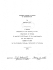### Филолошки факултет „Блаже Конески“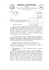### KRONOS Voice Name List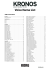### Christian Mio Loclair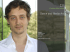### Bewachte Wohnkomplexe und "die europäische Stadt" : eine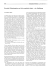### 1 - The Fibonacci Quarterly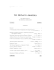### colloid grease mill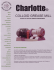### tại đây - thongke.info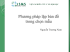### ASYMPTOTIC NORMALITY OF DEGREE COUNTS IN A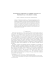### The role of the hospital pharmacy in the storage and supply of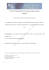### Specialty Dry Ink Business Development Guide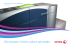### Scarica PDF - Istituto Poligrafico e Zecca dello Stato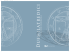### Nonparametric Estimation of Density Function Projektbereich A Discussion Paper No. A{544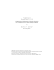### Full text - The Fibonacci Quarterly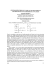### nms beverage guide - National Marine Suppliers